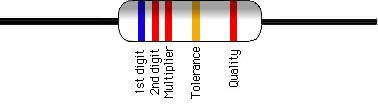# How to read Resistor Color Codes

### Color Code:

 Black Brown Red Orange Yellow Green Blue Violet Gray White 0 1 2 3 4 5 6 7 8 9

##### How to read the color code

• First find the tolerance band, it will typically be gold ( 5%) and sometimes silver (10%)
• Starting from the other end, identify the first band:
• Write down the number associated with that color
• In this example it is blue or 6
• Now 'read' the next color:
• In the example it is red so write down a '2' next to the six. (you should have '62' so far.)
• Now read the third or 'multiplier' band:
• Write down that number of zeros
• In this example it is two so we get '6200' or '6,200'
• If the 'multiplier' band is Black (for zero) don't write any zeros down
• If the 'multiplier' band is Gold move the decimal point one to the left
• If the resistor has one more band past the tolerance band it is a quality ban
• Read the number as the '% Failure rate per 1000 hour'
• This is rated assuming full wattage being applied to the resistors.
• To get better failure rates, resistors are typically specified to have twice the needed wattage dissipation that the circuit produces) 1% resistors have three bands to read digits to the left of the multiplier
• They have a different temperature coefficient in order to provide the 1% tolerance